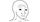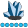somebody
Lapis Lazuli | Level 10

## Vector Autoregressive models

I am estimating a VAR model with only 2 variable X1 and returns for stocks. Currently, I estimate using PROC VARMAX with a BY statement. I then get the averages across all stocks. My code is :

``````*Estimating the model;
dm 'odsresults; clear';ods _all_ close; run; quit;
ods output ParameterEstimates=parameterEstimates covInnovation=cov ARRoots=ARRoots;;
proc varmax data=test_sample ;
by stock;
model X Return /p=10 method=LS lagmax=10 print=(estimates covpe roots);
run;quit;
options nomprint;

* Extract the relevant variances and covariances;
data cov1 (keep=stock var_X); set cov; where variable="X"; var_X=X; run;
data cov2 (keep=stock var_return); set cov; where variable="return"; var_return=return; run;
data cov3 (keep=stock covar_X_return); set cov; where variable="X"; covar_X_return=return; run;
``````

I then use these cov datasets as well as the parameterestimates dataset to compute the Impulse Response function for each stock ( I create a random shock and then compute the dynamics with 100 time period). I then get the average across all stocks to get the final numbers for the model. I dont post the code here because I dont want to confuse the readers.

My questions are:

1. Is there a quick step to estimate the VAR across multiple stocks (or groups such as stock-date)?

2.  what is a quick way to produce the Impulse Response Function? That is 1 set of results for multiple stocks, NOT for each individual stock because I want to estimate for the market as a whole. Note that I would want to see the dynamics for a window that is longer than the number of lag used in the model.

Discussion stats
• 0 replies
• 445 views
• 0 likes
• 1 in conversation